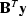Next: Solver Up: A deconvolution example Previous: Operator

## Deconvolution

The code fragment tcaiDeconis the main routine of the deconvolution problem. The class instantiates two Rsf objects from corresponding data files. One contains the blurred time series, the other one the blurring filter. Next, the program instantiates a transient convolution operator, convB. Since the conjugate-gradient solver requires a positive-definite operator, we recast the example's operator and vectors according to the normal equationsThe convolution's convB.adjoint() method yields the adjoint operator. Jest's CompoundLinearOperatorAdjoint class chains the forward and adjoint of conv to implementin a single operator convBn. Jest provides a family of such compound operator classes. To create a starting vector for the solution, we request a new member from the domain of the operator. The values of the vector elements default to zero. Finally, we instantiate a simple CG solver that estimates the unblurred image. The integer argument at the solver's instantiation is the number of iterations and the arguments of the solve method are the elements of the normal equation: the compound operator, the known data vector, and the initial guessof the unknown model vector. The solver updates the vectorto its estimate of the model. The last line of the deconvolution routine writes the estimated image to the system's standard output stream. Figure 1 shows the inputs and the result of the inversion.
package conv.decon;
import jam.vector.*;
import juice.solver.*;
import juice.operator.*;
import rsf.vector.*;
import conv.convt.*;
/**
* I solve y = B x for x.                                   <BR>
* y is a    known time series                      (trace).<BR>
* B is a    known convolution operator of the blur (wavlt).<BR>
* x is an unknown relectivity.                             <BR>
* This creates the normal equations: y_n = B'y and B_n = B'B and
* calls a CGSolver to solve y_n = B_n x, respectively B'y = B'B x.
*/
public class TcaiDecon {
public static void main(String[] args) {
Rsf  yy = RsfFactory.newRsf("./trace.H");      // read   y
Rsf  bb = RsfFactory.newRsf("./wavlt.H");      // read   b
Tcai convB = new TcaiFactory(bb).              // create B
getTcaiFromOutputSpace((RsfSpace) yy.getSpace());
Vector     yn = convB.adjoint().image(yy);     // create yn = B'y
CompoundLinOpAdj convBn =                      // create Bn = B'B